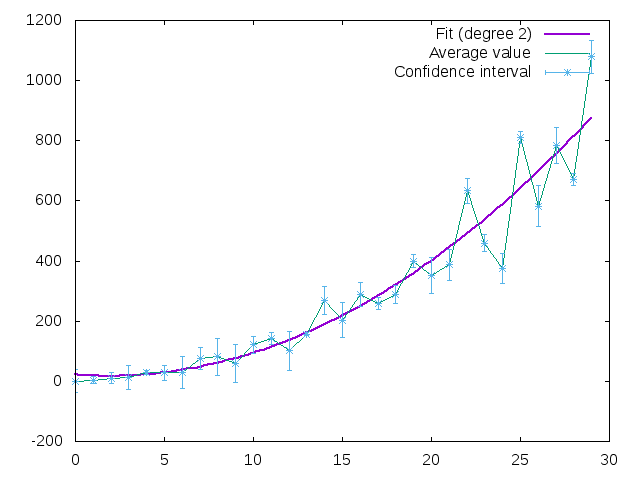### Fitting data with formula¶

Gnuplot is capable to fit given data with functions given as formulas. This notebook contain several examples about how to fit data with GnuplotRB.

First lets prepare some data:

In :
require 'daru'
require 'gnuplotrb'
include GnuplotRB
include Fit

rows = (1..30).map do |i|
[i**2 * (rand(4) + 3) / 5, rand(70) + 1]
end
df = Daru::DataFrame.rows(rows, order: [:Value, :Error], name: 'Confidence interval')

random_points = Plot.new(
[df[:Value], with: 'lines', title: 'Average value'],
[df, with: 'err']
)

Out:

And now lets try to fit it with some function.

First parameter of #fit is data (you can use here Datablock, Dataset or any other object out of which Datablock may be constructed). Special options are function and initials, other options (such as using) may be found in gnuplot docs. Be careful: you should give initial value for each coefficient used in formula.

Return value is the following hash:

{
:formula_ds => #<dataset with formula which fits given data>,
:coefficients => #<hash of calculated coefficients>,
:deltas => #<hash of possible deltas for coefficients>,
:data => #<given data>
}
In :
some_poly = fit(df, function: "a*x**3 + b*x + c", initials: {a: 1, b: 1, c: 1})
some_poly[:coefficients]

Out:
{:a=>0.0271674, :b=>8.64614, :c=>-6.69375}
In :
some_poly[:formula_ds].data

Out:
" 0.0271674*x**3 + 8.64614*x + -6.69375 "
In :
some_poly[:formula_ds]

Out:
In :
random_points << some_poly[:formula_ds]

Out:

#### Default fittings¶

Polynomial:

In :
poly = fit_poly(df)

Out:

Be default polynomial degree is 2 but you can set it:

In :
frames = (2..9).map { |i| random_points.add_dataset(fit_poly(df, degree: i)[:formula_ds].options(title: "Fit (degree #{i})", lw: 2)) }
Animation.new(*frames, animate: { delay: 150 } )

Out:You can also fit data with exp, sin and log like this:

In :
exp = fit_exp(df)

ranged_poly = fit_poly(df, degree: 4, term_options: { xrange: 0..15})